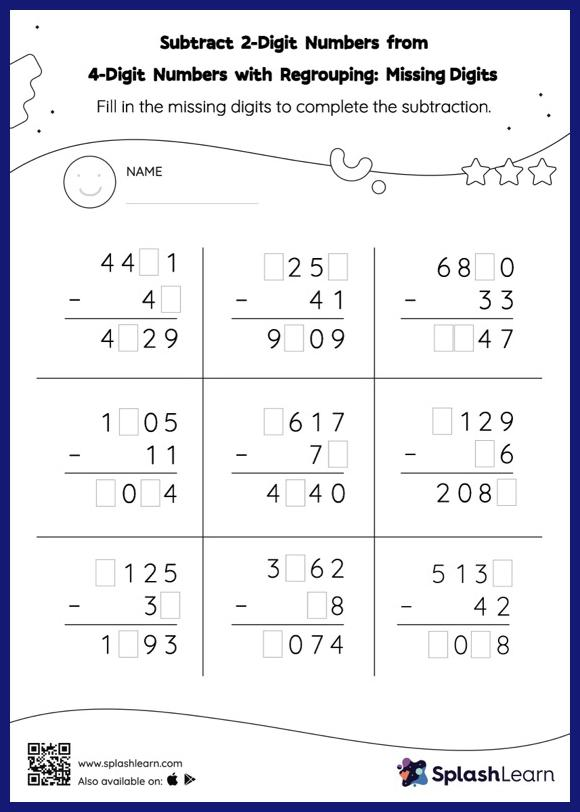# Subtract 2-Digit Numbers from 4-Digit Numbers with Regrouping: Missing Digits Worksheet

Home > Subtract 2-Digit Numbers from 4-Digit Numbers with Regrouping: Missing DigitsThis subtract 2-digit numbers from 4-digit numbers with regrouping worksheet consists of a set of questions on subtraction, which help students develop fluency. In this worksheet, students practice solving problems using the column method. This method is especially helpful with problems involving bigger multi-digit numbers as the format provides an easy structure to follow.